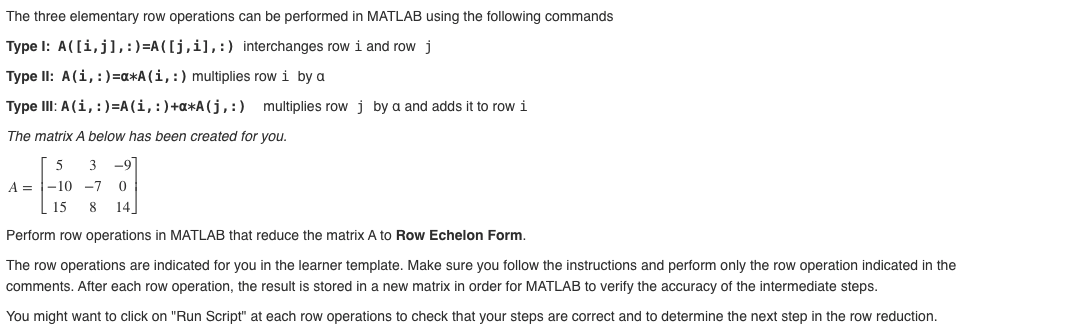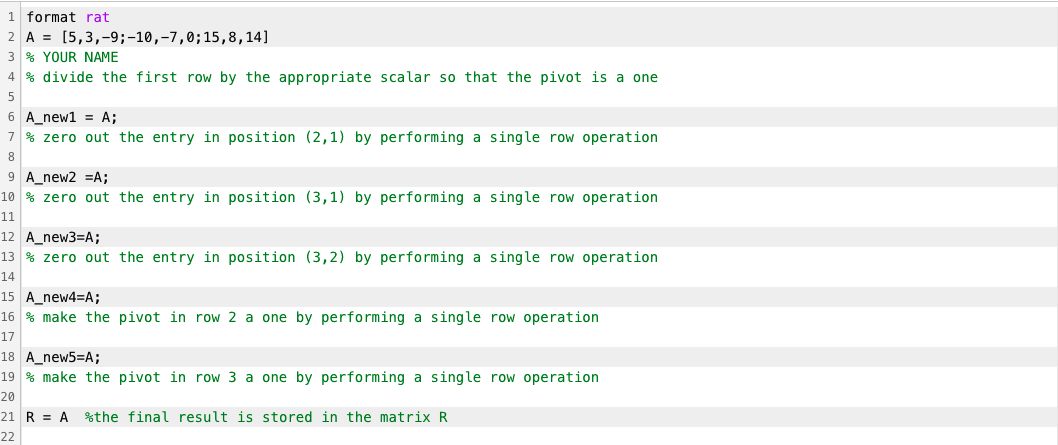# Question Using MATLAB, The three elementary row operations can be performed in MATLAB using the following commands Type I: A([i,j], :)=A([j,i],:) interchanges row i and row j Type II: Ali, :)=a*A(i, :) multiplies row i by a Type III: A(i, :)=A(i, :)+a*A (),:) multiplies row j by a and adds it to row i The matrix A below has been created for you. -9 5 3 A=-10 -7 15 8 0 14 Perform row operations in MATLAB that reduce the matrix A to Row Echelon Form. The row operations are indicated for you in the learner template. Make sure you follow the instructions and perform only the row operation indicated in the comments. After each row operation, the result is stored in a new matrix in order for MATLAB to verify the accuracy of the intermediate steps. You might want to click on "Run Script" at each row operations to check that your steps are correct and to determine the next step in the row reduction. 1 format rat 2 A = [5,3,-9;-10,-7,0; 15,8,14] 3 % YOUR NAME 4 % divide the first row by the appropriate scalar so that the pivot is a one 5 6 A_new1 = A; 7 % zero out the entry in position (2,1) by performing a single row operation 8 9 A_new2 =A; 10 % zero out the entry in position (3,1) by performing a single row operation 11 12 A_new3=A; 13 % zero out the entry in position (3,2) by performing a single row operation 14 15 A_new4=A; 16 % make the pivot in row 2 a one by performing a single row operation 17 18 A_new5=A; 19 % make the pivot in row 3 a one by performing a single row operation 20 21 R = A %the final result is stored in the matrix R 22